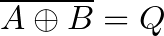# XNOR Gate – Logic Gates Tutorial

An XNOR gate is a logic gate where the output goes HIGH (or “1”) only if both its inputs are equal. So if the inputs A and B are both HIGH or both LOW, the output Q will be HIGH.

The logic or Boolean expression for an XNOR gate iswhich means that:

If A and B are the same, then Q is true

## Truth Table

XNOR gates commonly only have two inputs. It will only give out a HIGH or logic “1” if both its inputs are equal. So the inputs must both be HIGH or both be LOW for the output to become HIGH.

## IC Alternatives with XNOR Gates

If you want to experiment and build circuits with XNOR gates, you’ll find them in both the 4000 IC series and the 7400 IC series:

• 4077: Four XNOR gates
• 74HC266: Four XNOR gates (HC is the family, can also be LS/HCT/…)

These should all be available as hobbyist-friendly through-hole chips. Just make sure you buy the DIP package version.

## Example Circuit: XNOR Gate Edge Detection

This circuit detects if a signal is going from LOW to HIGH or from HIGH to LOW. The Light-Emitting Diode (LED) on the output turns on for a short amount of time when an edge is detected.

For fast-switching signals, you’d need lower values for R1 and C1, and the LED would be ON for such a short time that it wouldn’t make sense. But instead, it would make sense to for example read the output into a microcontroller, or use a counter to count the edges.